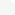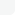## Categories# Video Training →Fundamental Data Structures & Algorithms using C language

Genre: eLearning | MP4 | Video: h264, 1280x720 | Audio: aac, 48000 Hz
Language: English | VTT | Size: 5.14 GB | Duration: 15h 42m

What you'll learn
Recursion, Stack, Polish Notations, infix to postfix, FIFO Queue, Circular Queue, Double Ended Queue, Linked List - Linear, double and Circular - all operations, Stack and Queue using Linked List
What is stack, algorithms for Push and Pop operation. Implementation of Stack data structure using C.
Using Stack - checking parenthesis in an expression
Using Stack - Understanding Polish notations, algorithm and implementation of infix to postfix conversion and evaluation of postfix expression
What is a FIFO Queue, understanding Queue operations - Insert and delete, implementing FIFO Queue
Limitations of FIFO queue, concept of Circular Queue - Implementation of Circular queue.
Concept of Double ended queue, logic development and implementation of double ended queue.
Singly Linked List - developing algorithms for various methods and then implementing them using C programming
Doubly Linked List - developing algorithm of various methods and then implementing them using C programming
Circular Linked List - developing algorithm of various methods and then implementing them using C programming
How to estimate time complexity of any algorithm. Big Oh, Big Omega and Big Theta notations.
Recursion, concept of Tail recursion, Recursion Vs Iteration.
Binary Tree, definition, traversal (in-order, pre-order and post-order), binary search tree, implementation.
Heap - concept, definition, almost complete binary tree, insertion into heap, heap adjust, deletion, heapify and heap sort.
Requirements
Student should be conversant with basic C programming.
Description
You will learn the following in this course: (All implemented using C programming)
Fundamental of Data Structure concept
Why we need Data Structures
Stack - Idea, definition, algorithm, implementations.
Using Stack - Parenthesis checking, Polish Notation, Infix to postfix conversion and evaluation.
FIFO Queue - Idea, definition, algorithm, implementation.
Circular Queue using array - Idea, definition, algorithm, implementation.
Double ended queue using array - Idea, definition, algorithm, implementation.
Linked List - Idea, definition, why we need linked list. Comparison with array.
Singly Linked List - Development of algorithm for various operations and then Implementation of each of them
Creating Stack and Queue using Singly Linked list - Implementation.
Doubly Linked List - Idea, definition, algorithm of various operations and implementations.
Circular Linked List - Idea, definition, algorithm and implementations.
14. Calculating efficiency of algorithms, Worst Case (Big Oh), Average Case (Big Theta) and Best case (Big omega) complexities. How to calculate them for different algorithms.
15. Binary Searching
16. Recursion in detail. Example program using recursion and the critical comparison between Recursive approach and Iterative approach of problem solving.
17. Binary Tree, definition, traversal (In, Pre and Post Order), Binary Search Tree implementation.
18. Heap data structure, definition, heap insertion, deletion, heap adjust, Heapify and heap sort.
Who this course is for:
Students who want to prepare themselve for interview of top companies like Google, Amazon or Microsoft.
Students who are looking forward to be an efficient programmer, who are having data structures in their syllabus.
Students who wants to have in dept knowledge about the Stack, Queue and Linked List, Efficiency of Algorithm, Binary Tree, Heap

Homepage
``https://www.udemy.com/course/data-structures-stack-queue-linkedlist/``

## Related News## Information

Users of Guests are not allowed to comment this publication.